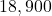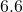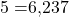## Cleo buys a new car for $18,900. The simple interest rate is 6.6% and the amount of loan (plus simple interest) is repayable in 5 years. Wha Question Cleo buys a new car for$18,900. The simple interest rate is 6.6% and the amount of loan (plus simple interest) is repayable in 5 years. What is the total amount that must be repaid?

in progress 0
2 months 2021-08-28T07:39:55+00:00 1 Answers 0 views 0

calculated×